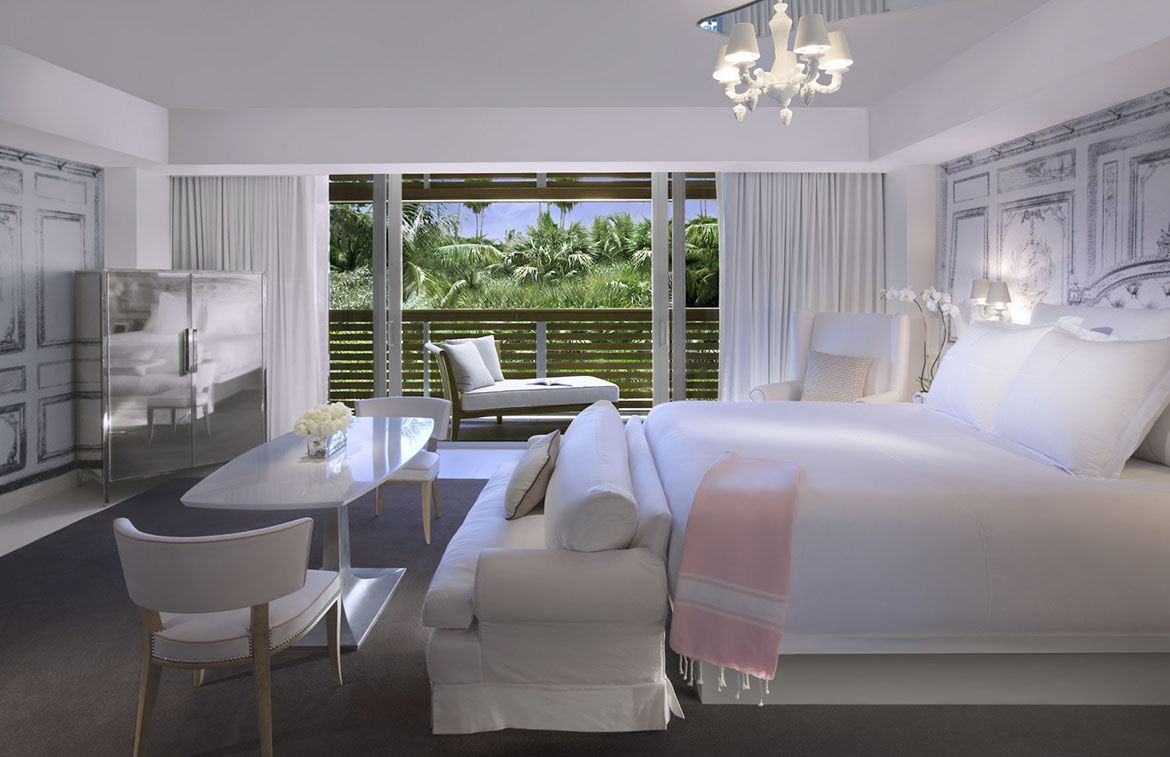# Villa Bedroom

## Villa BedroomThis is sample text and this is where you would describe the project. This is sample text and this is where you would describe the project. This is sample text and this is where you would describe the project. This is sample text and this is where you would describe the project. This is sample text and this is where you would describe the project. This is sample text and this is where you would describe the project. This is sample text and this is where you would describe the project. This is sample text and this is where you would describe the project. This is sample text and this is where you would describe the project.

This is sample text and this is where you would describe the project. This is sample text and this is where you would describe the project. This is sample text and this is where you would describe the project.